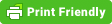# Getting the Correct Notebook Tab Across Platforms in wxPython

I was recently working on a GUI application that had a `wx.Notebook in it. When the user changed tabs in the notebook, I wanted the application to do an update based on the newly shown (i.e. selected) tab. I quickly discovered that while it is easy to catch the tab change event, getting the right tab is not as obvious.`

``` This article will walk you through my mistake and show you two solutions to the issue. Here is an example of what I did originally: # simple_note.py import random import wx class TabPanel(wx.Panel): def __init__(self, parent, name): """""" super().__init__(parent=parent) self.name = name colors = ["red", "blue", "gray", "yellow", "green"] self.SetBackgroundColour(random.choice(colors)) btn = wx.Button(self, label="Press Me") sizer = wx.BoxSizer(wx.VERTICAL) sizer.Add(btn, 0, wx.ALL, 10) self.SetSizer(sizer) class DemoFrame(wx.Frame): """ Frame that holds all other widgets """ def __init__(self): """Constructor""" super().__init__(None, wx.ID_ANY, "Notebook Tutorial", size=(600,400) ) panel = wx.Panel(self) self.notebook = wx.Notebook(panel) self.notebook.Bind(wx.EVT_NOTEBOOK_PAGE_CHANGED, self.on_tab_change) tabOne = TabPanel(self.notebook, name='Tab 1') self.notebook.AddPage(tabOne, "Tab 1") tabTwo = TabPanel(self.notebook, name='Tab 2') self.notebook.AddPage(tabTwo, "Tab 2") sizer = wx.BoxSizer(wx.VERTICAL) sizer.Add(self.notebook, 1, wx.ALL|wx.EXPAND, 5) panel.SetSizer(sizer) self.Layout() self.Show() def on_tab_change(self, event): # Works on Windows and Linux, but not Mac current_page = self.notebook.GetCurrentPage() print(current_page.name) event.Skip() if __name__ == "__main__": app = wx.App(False) frame = DemoFrame() app.MainLoop() This code works correctly on Linux and Windows. However when you run it on Mac OSX, the current page that is reported is always the tab that you were on before you selected the current page. It's kind of like an off-by-one error but in a GUI. After trying our a couple of ideas on my own, I decided to ask the wxPython Google group for help. They had two workarounds: Use GetSelection() along with the notebook's GetPage() method Use the FlatNotebook widget Using GetSelection() Using the event object's GetSelection() method will return the index of the currently selected tab. Then you can use the notebook's GetPage() method to get the actual page. This was the suggestion that Robin Dunn, the maintainer of wxPython, gave to me. Here is the code updated to use that fix: # simple_note2.py import random import wx class TabPanel(wx.Panel): def __init__(self, parent, name): """""" super().__init__(parent=parent) self.name = name colors = ["red", "blue", "gray", "yellow", "green"] self.SetBackgroundColour(random.choice(colors)) btn = wx.Button(self, label="Press Me") sizer = wx.BoxSizer(wx.VERTICAL) sizer.Add(btn, 0, wx.ALL, 10) self.SetSizer(sizer) class DemoFrame(wx.Frame): """ Frame that holds all other widgets """ def __init__(self): """Constructor""" super().__init__(None, wx.ID_ANY, "Notebook Tutorial", size=(600,400) ) panel = wx.Panel(self) self.notebook = wx.Notebook(panel) self.notebook.Bind(wx.EVT_NOTEBOOK_PAGE_CHANGED, self.on_tab_change) tabOne = TabPanel(self.notebook, name='Tab 1') self.notebook.AddPage(tabOne, "Tab 1") tabTwo = TabPanel(self.notebook, name='Tab 2') self.notebook.AddPage(tabTwo, "Tab 2") sizer = wx.BoxSizer(wx.VERTICAL) sizer.Add(self.notebook, 1, wx.ALL|wx.EXPAND, 5) panel.SetSizer(sizer) self.Layout() self.Show() def on_tab_change(self, event): # Works on Windows, Linux and Mac current_page = self.notebook.GetPage(event.GetSelection()) print(current_page.name) event.Skip() if __name__ == "__main__": app = wx.App(False) frame = DemoFrame() app.MainLoop() That was a fairly simple fix, but kind of annoying because it's not obvious why you need to do that. Using FlatNotebook The other option was to swap out the wx.Notebook for the FlatNotebook. Let's see how that looks: # simple_note.py import random import wx import wx.lib.agw.flatnotebook as fnb class TabPanel(wx.Panel): def __init__(self, parent, name): """""" super().__init__(parent=parent) self.name = name colors = ["red", "blue", "gray", "yellow", "green"] self.SetBackgroundColour(random.choice(colors)) btn = wx.Button(self, label="Press Me") sizer = wx.BoxSizer(wx.VERTICAL) sizer.Add(btn, 0, wx.ALL, 10) self.SetSizer(sizer) class DemoFrame(wx.Frame): """ Frame that holds all other widgets """ def __init__(self): """Constructor""" super().__init__(None, wx.ID_ANY, "Notebook Tutorial", size=(600,400) ) panel = wx.Panel(self) self.notebook = fnb.FlatNotebook(panel) self.notebook.Bind(wx.EVT_NOTEBOOK_PAGE_CHANGED, self.on_tab_change) tabOne = TabPanel(self.notebook, name='Tab 1') self.notebook.AddPage(tabOne, "Tab 1") tabTwo = TabPanel(self.notebook, name='Tab 2') self.notebook.AddPage(tabTwo, "Tab 2") sizer = wx.BoxSizer(wx.VERTICAL) sizer.Add(self.notebook, 1, wx.ALL|wx.EXPAND, 5) panel.SetSizer(sizer) self.Layout() self.Show() def on_tab_change(self, event): # Works on Windows, Linux and Mac current_page = self.notebook.GetCurrentPage() print(current_page.name) event.Skip() if __name__ == "__main__": app = wx.App(False) frame = DemoFrame() app.MainLoop() Now you can go back to using the notebook's GetCurrentPage() method. You can also use self.notebook.GetPage(event.GetSelection()) like you do in the other workaround, but I feel like GetCurrentPage() is just more obvious what it is that you are doing. Wrapping Up This is one of the few times that I was caught by a strange gotcha in wxPython. You will come across these sorts of things from time to time when you are programming code that is meant to run across multiple platforms. It's always worth checking the documentation to make sure you're not using a method that is not supported on all platforms. Then you will want to do some research and testing on your own. But once you have done your due diligence, don't be afraid to ask for help. I will always seek assistance over wasting many hours of my own time, especially when it is something like this where my solution worked in 2 out of 3 cases.```
` `
``` ```
``` Post navigation ← Sending email with EZGmail and Python Book Review: Practical Python and OpenCV → ```